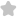SELECT THE CORRECT ALTERNATIVES (ON...
Question# SELECT THE CORRECT ALTERNATIVES (ONE OR MORE THAN ONE CORRECT ANSWERS The equation whose roots are seca a & coseca, can be (A) 2x² - x-1=0 (B) x² - 3x + 3 = 0 e 54+G=0 12. If cos a is a root of the equation 25x + 5x - 12 = 0, - 1 JEE/Engineering Exams Maths SolutionShare694.0 (1 ratings)Q Tru rorts sec"d ( operatorname{cosec}^{2} alpha ) [ begin{array}{l} a=sec ^{2} alpha b=operatorname{cosec}^{2} alpha end{array} ] As we know theet the equation form by two roots ( i delta ) ( x^{2}-(a+b) x+a b=0 ) ( x^{2}-left(sec ^{2} alpha+cos e c^{2} alpharight) x+sec ^{2} alpha cdot operatorname{cosec}^{2} alpha=0 ) [ sec alpha=sqrt{a} rightarrow frac{d}{d}left|sqrt{a}_{1}rightrangle sqrt{a+1} ] ( sec ^{2} alpha=a=1 ) ( 6-1 ) ( operatorname{cosec}^{2} alpha=b=2 quad sqrt{b}=sqrt{a+1} quad sqrt{b-1}=1 ) ( x^{2}-(1+2) x+2=0 quad frac{b=a+1}{2-a=1} )694.0 (1 ratings) Rate SolutionShareQuick and Stepwise Solutions Just click and SendOVER 20 LAKH QUESTIONS ANSWERED Download App for Free